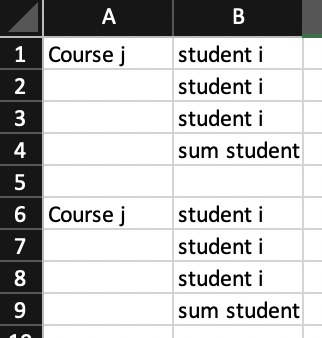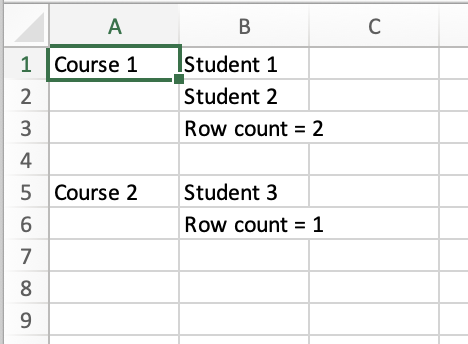# Sum of JuMP.value

Hello,

I would like to display in an excel document the sum of students assigned for each course:

``````if JuMP.value.(x[i,j])==1
``````

for each j, display the sum of x[i,j]

Could someone help me?

1 Like
``````students_in_each_course = zeros(J)
for j in 1:J
for i in 1:I
if value(x[i,j]) == 1
students_in_each_course[j] += 1
end
end
end

println(students_in_each_course)
``````

Thank you very much!

Do you have any idea how I could display my solution in the following form:
from Julia in excel?for the moment I have this:

``````XLSX.openxlsx("choix élèves julia.xlsx", mode="rw") do xf
sheet["A1", dim=2] = collect(1:3)
``````
1 Like

if value(x[i,j]) == 1

Never do this. Even if `x` is integer or binary, the result is not guaranteed to be exactly one. Do instead

``````if isapprox(value(x[i, j]), 1; atol = 1e-6)  # <-- or some other tolerance
``````

See this part of the docs: Getting started with Julia · JuMP

However, since it seems you probably are using binary variables, you can also do:

``````students_in_each_course = [sum(value.(x[:, j])) for j in 1:J]
``````

Do you have any idea how I could display my solution in the following form

What, exactly, do you want to represent? The A column is the name of the course, the B column is the name of the students assigned to each course, and the last row of each course is the number of students?

I’d do something like this:

``````using HiGHS
using JuMP
using XLSX

function main(students, courses)
model = Model(HiGHS.Optimizer)
@variable(model, x[students, courses], Bin)
@constraint(model, [i in students], sum(x[i, :]) == 1)
@constraint(model, [j in courses], sum(x[:, j]) >= 1)
optimize!(model)
XLSX.openxlsx("output.xlsx", mode = "w") do io
end
row = 1
for course in courses
sheet["A\$row"] = "Course \$course"
count = 0
for student in students
if isapprox(value(x[student, course]), 1; atol = 1e-6)
sheet["B\$row"] = "Student \$student"
row += 1
count += 1
end
sheet["B\$row"] = "Row count = \$count"
end
row += 2
end
end
return
end

students, courses = 1:3, 1:2
main(students, courses)
``````

which yieldsIt might not be exactly what you want, but hopefully it points you in the right direction.

1 Like